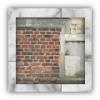#### You may also like### Rule of Three

If it takes four men one day to build a wall, how long does it take 60,000 men to build a similar wall?### Square Pegs

Which is a better fit, a square peg in a round hole or a round peg in a square hole?### How Big?

If the sides of the triangle in the diagram are 3, 4 and 5, what is the area of the shaded square?

# Mixing Paints

##### Age 11 to 14Challenge Level

Why do this problem?

This problem requires students to think very carefully about what to use as their basic unit and how to combine ratios. Many students (and teachers!) initially try combining $1:3$ and $1:7$ and assume that the $1$ in each case represents the same quantity of paint, rather than considering a volume of paint and the proportion that is made up of each shade. This offers a great opportunity for discussion about the importance of checking assumptions carefully!

As students work towards generalisation, they will need to show resilience and perseverance to succeed.

Possible approach

Introduce the two paints from the problem:

• Paint A is made up from red and white paint in the ratio 1:3
• Paint B is made up from red and white paint in the ratio 1:7

"What would the ratio of red to white paint be if the painter mixed one can of Paint A and one can of Paint B?" Give students some thinking time, then invite them to check that they agree with their partner, and finally share answers with the whole class.

Some students will initially give the answer $1:5$, making the argument that if A is $1:3$ and B is $1:7$, A+B is $2:10$ which simplifies to $1:5$. One way to help them to spot their error is to choose a particular size of paint can (1 litre, perhaps):
1 litre of Paint A: 250ml red, 750ml white.
1 litre of Paint B: 125ml red, 875ml white.
Mix them together: 375ml red, 1625ml white, which is in a ratio $3:13$.

Once everyone is happy, invite students to choose some combinations of paints A and B and work out the ratios. Then, once they have had a chance to explore, challenge them to find ways of combining A and B to give the ratios $1:4$, $1:5$, and $1:6$.

If computers/tablets are available, students could use the interactivity from the problem to experiment or to check their answers.

Some students may wish to practise any strategies they have developed by working on paint sets C and D and using the second interactivity. Others might want to get started on the final challenge in the problem: can they come up with a method to combine two paints made up in the ratios 1:x and 1:y and turn them into paint made up in the ratio 1:z?

Allow some time at the end of the lesson for students to share any strategies they came up with for finding particular targets, and if appropriate, to look at a general strategy.

### Key questions

Why might it be sensible to consider cans that hold 8 litres?
$1:3 = 2:6 = 3:9 = ...$ Which of these ratios might be the most useful when mixing paint A with paint B?
What would the paint colours look like? Which would be darkest? Which would be lightest?
Is it possible to make paint D ($1:9$) from paint A and paint B?

### Possible support

Students could use different coloured counters or multilink cubes to represent the cans of paint, being sure to use the same total for each can of paint, or use diagrams by shading squares on squared paper.

### Possible extension

Mixing More Paints invites students to find a strategy for making any ratio.